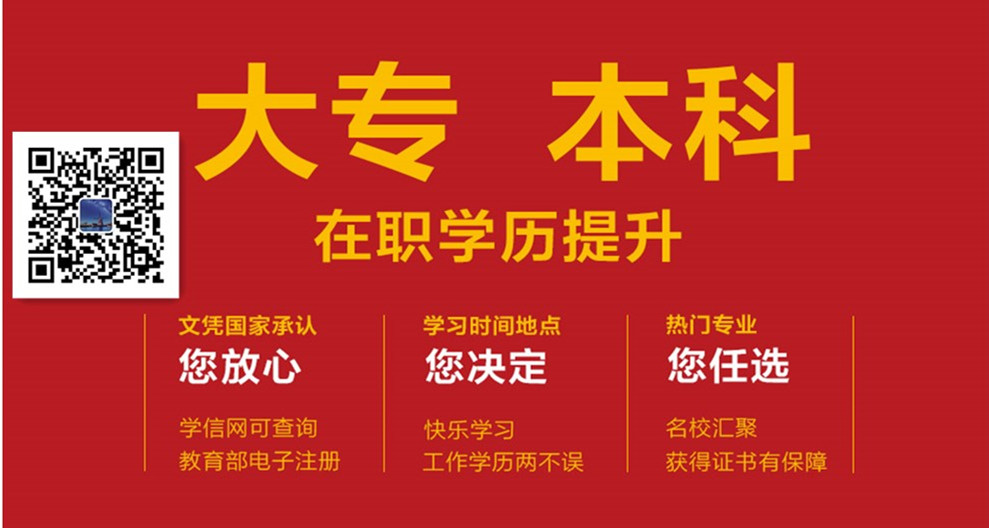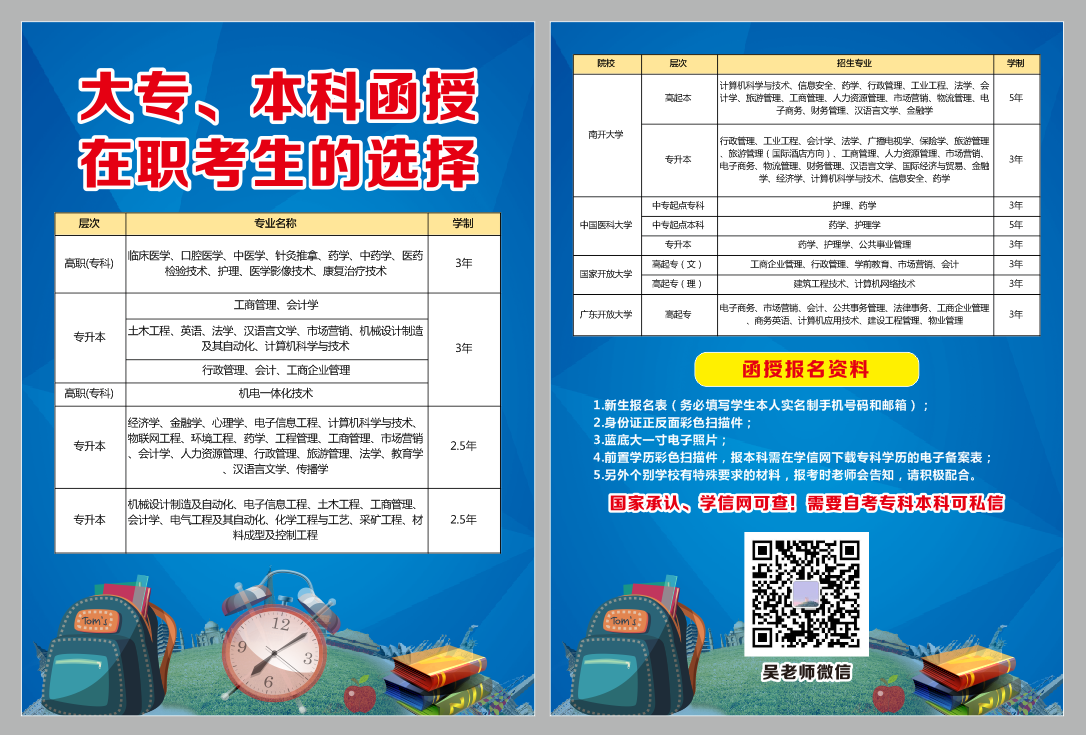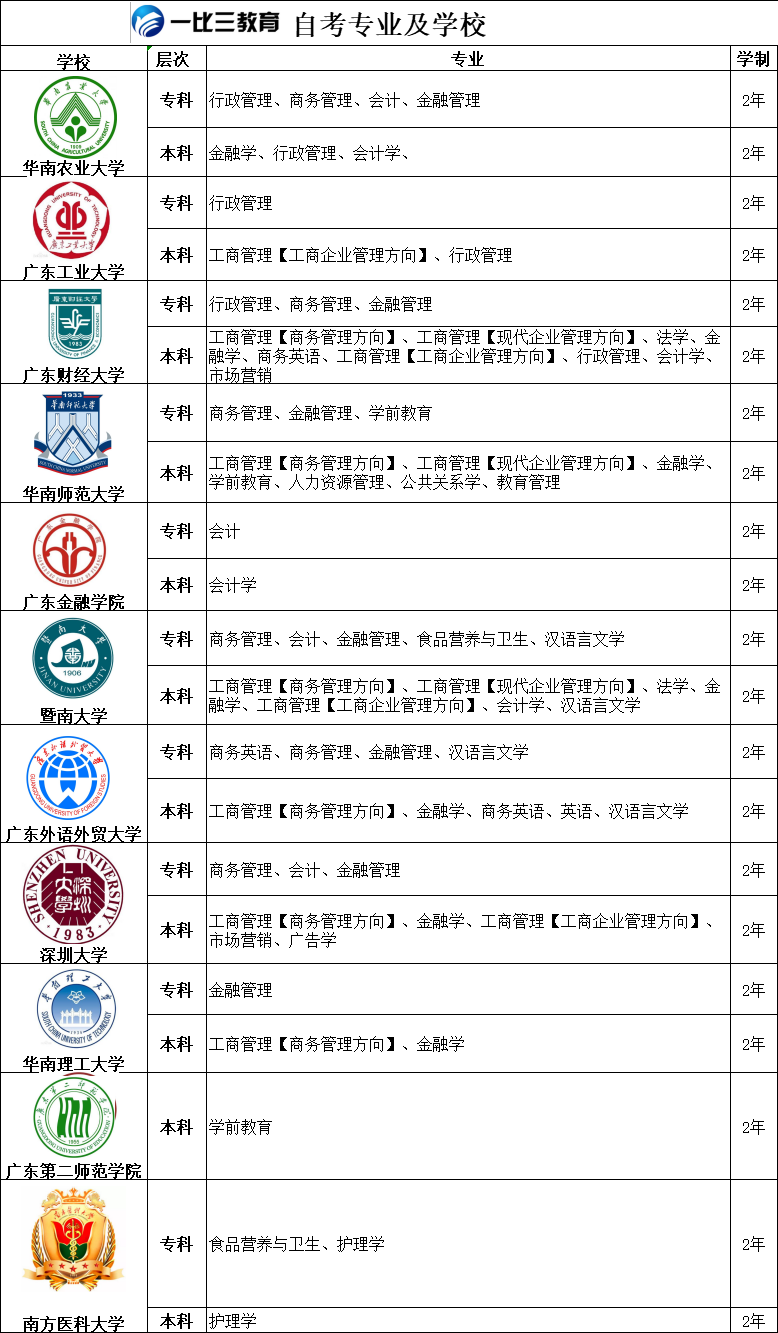• 专科
• 本科
• 考研
• 资格证书
教师资格证
会计职称考试
一级消防工程师
一级造价师
• 专插本

# 2019年广东成考高起点《数学》真题及答案解析

【参考答案（部分）】

2019年成人高等学校招生全国统一考试

本试卷分第I卷(选择题)和第II卷(非选择题)两部分,共4页。满分150分考试时间120分钟。

第I卷(选择题，共85分)

一、选择题:本大题共17小题，每小题5分，共85分。在每个小题给出的四个选项中，选出一项符合题目要求的。

1.设全集U=(1,2,3,4},集合M=(3.4],则CJM =

A.{2,3}B.[2,4}C{L4}D.(1.2}

[答案] D

[解析]求补集，是集合缺少的部分，应该选D2.函数y = cos4x的最小正周期为

A.π/4

B.π/2

C.π

D.π

[答案] B

3.设甲: b=0;乙:函数y= kx + b的图像经过坐标原点，则

A.甲是乙的充分条件但不是必要条件

B.甲是乙的必要条件但不是充分条件

C.甲是乙的充要条件

D.甲既不是乙的充分条件也不是乙的必要条件[答案] C

[解析]本题考查了充分条件和必要条件的知识点，

5.函数y= 根号1- x石的定义域是

A. {x|x≥-1}

B. {x|x≤ 1}

C. {x|x≤-1}

D. {xI-1≤x≤1}

[答案] D

[解析] 1-x°≥0时，原函数有意义，即x°≤1即{x|-1≤x≤1}

6.设0

A.1<2*x<2

B. 0<2*< 1

C. log1/2x< 0

D. log:x > 0

[答案] A

7.不等式|x+1/2|>1/2的解集为

A. {x|-1

B. {x|x>0或x<-1}

C. {x|x>-1}

D. {x|x< 0}

[答案] B

8.甲、乙、丙、可4人排成一行，其中甲、乙必须排在两端，则不同的排法共有

A.2种B.4种C.8种D，24种

[答案] B

9.若向量a=(1,1), b=(1,-1)，则≌a-gb=A. (-1,2) B. (1,-2)C. (1,2)

D. (-1,-2)

[答案] A

10.log21 + 161/2+(-2)°=

A.5B.4C.3D.2

[答案] B

[解析] logg1+ 161+(-2)°=0+4+1=5

11.函数y=x≥- 4x- 5的图像与x轴交于A、B两点，则|AB|=

A.3B.4C.5D.6

[答案] D

[解析] x好-4x-5= 0解得x=-1或x= 5,则A、B两点距离lABI= 612.下列函数为奇函數的是A. y=-2x+3B. y=-EC. y=x2-3D. y= 3cosx[答案] B

[解析]满足f(-x)=-f6)为奇函数

13.双曲线”-些= 1的焦点坐标为

A. (-5,0)，。 (5,0)

B. (-V70)，(V70)

C. (0,-5)，60,5)

D. (0，一7)，(0,V7)

[答案] A

[解析]显然x2的系数大于0，则焦点在x轴。又c=√a°+b=V9+ 16= 5,则焦点坐标为(-5,0)，(5,0)

以下题目缺少题干，答案仅供参考14.B15.B .16.A .17.C

二、填空题:本大题共4小题，每小题4分，共16分。

【参考答案（部分）】

2019年成人高等学校招生全国统一考试

本试卷分第I卷(选择题)和第II卷(非选择题)两部分共4页。满分150分,考试时间120分钟。考试结束后，将本试卷和答题卡- -并交回。注意事项:

第I卷(选择题，共85分)

一、选择题:本大题共17小题，每小题5分，共85分。在每个小题给出的四个选项中，选出- -项符合题目要求的。

1.设全集U=({,23.4),集合M=(3,4,则CuM =

A.{2,3}B.{2,4]}C(1,4}D.(1,2}

[答案] D .

[解析]求补集，是集合缺少的部分，应该选D

2.函数y = cos4x的最小正周期为

A.I

B，π

D.2π

[答案] c

[解析]本题考查了三角函数的周期的知识点最小正周期.设用: b=0;乙:函数y= kx + b的图像经过坐标原点，则

A.甲是乙的充分条件但不是必要条件

B.用是乙的必要条件但不是充分条件

C.甲是乙的充要条件

D.甲既不是乙的充分条件也不是乙的必要条件

[答案] C

[解析]本题考查了充分条件和必要条件的知识点，

4.已知tana=1/2，则tan(a+π/4)=

A.-3

B.一1/3

c.1/2

D.3

[答案] D

5.函数y=√1-x2“的定义域是

A. {x|x≥-1}

B. {xIx≤1}C. {x|x≤-1}

D. {x|-1≤x≤1} .[答案] D

[解析] 1-x°≥0时，原函数有意义，即x°≤1即(x1-1≤x≤1}6.设0

物

D. log;x> 0[答案] B

[解析] 1<2*<2，logx> 0，logax<0

7.不等式|x +第>当的解集为A. {x|-1- -1] ，

C. {1>0或x<-1}D. {xkx<0}[答案] C

[解析] |x+当≥当解得x+ξ<←或x+>即{x|x>0或x<-1}

8.甲、乙、丙、丁4人排成一行，其中甲、乙必须排在两端，则不同的排法共有

A.3种

B. 8种

C.4种

D.24种

[答案] C

[解析]甲乙站在两边，有2种排法，丙丁站在中间有2种排法，总计: 2*3=4.

9,若向量a=(1,), b=(1,-1), 则1/2a-3/2b=;

A. (-1,2)

B. (1,-2)

C. (1,2)

D. (-1,-2)

【答案】 A

11,y=x2- 4x- 的图像与x轴交于A.B两点，则丨AB 丨=

A.3

B 4

C.5

D.6

(答案) D

12【答案】c

13【答案】b

14.若直线mx +y-1= 0与直线4x+ 2y+1= 0平行，则m=

A. -1

B.0

C.1

D.2

[答案] D

[解析]直线平行，斜率相等

15.在等比数列中，若a4a5= 6，则a2a3a6a7,=

A.36

B.24

C. 12

D.6

[答案] A

[解析]等比数列性质，下角标之和相等，乘积相等，则asag= azay= azae,则azazagaz =36

16.已知函数f(x)的定义域为R，且f(2x)=4x+ 1,则f(1) =

A.5

B.3

C.7

[答案] B

[解析]令x =则f(2x)=4x + 1变为f(2x号)=4x2+ 1=3

17.甲、乙各独立地射击一次，己知甲射中10环的概率为0.9,乙射中10换的概率为0.5，则甲、乙都射中10环的概率为

A.0.45

B.0.25

C.0.2

D.0,75

[答案] A

[解析]甲、乙射击是独立的，则甲、乙都射中10环的概率为0.9*0.5=0.45以下题目缺少题干，答案仅供参考

二、填空题:本大题共4小题，每小题4分，共16分。18， 19.0 20， 4 21，0.7

三、计算题:本大题共4小题，22-24 每小题12分，25题13分，共49分。

22.缺少题干，仅供参考

S2o= 20a1 +- d=-55

23.AB= V6

24.缺少题干，仅供参考

函数在(-∞,-2)，(-V2, +oo)单增，(-√2,√2)单减

函数在x=-VZ处取得极大值为f(-v②)= 8√2+ 1

函数在x = V2处取得极小值为f(v2)=-8√2+ 1

已整理，更新上传中......联系方式